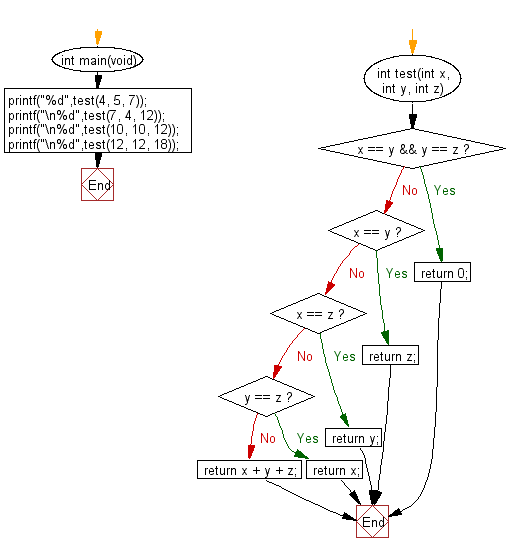﻿ C Program: Sum 3 integers, if 2 are same return third# C Exercises: Compute the sum of three given integers. If the two values are same return the third value

## C-programming basic algorithm: Exercise-29 with Solution

Write a C program to compute the sum of three given integers. If the two values are same return the third value.

C Code:

``````#include <stdio.h>
#include <stdlib.h>

int main(void){
printf("%d",test(4, 5, 7));
printf("\n%d",test(7, 4, 12));
printf("\n%d",test(10, 10, 12));
printf("\n%d",test(12, 12, 18));
}
int test(int x, int y, int z)
{
if (x == y && y == z) return 0;
if (x == y) return z;
if (x == z) return y;
if (y == z) return x;
return x + y + z;
}
``````

Sample Output:

```16
23
12
18
```

Pictorial Presentation:Flowchart:C Programming Code Editor:

What is the difficulty level of this exercise?

Test your Programming skills with w3resource's quiz.

﻿

## C Programming: Tips of the Day

Why doesn't a+++++b work?

printf("%d",a+++++b); is interpreted as (a++)++ + b according to the Maximal Munch Rule!.

++ (postfix) doesn't evaluate to an lvalue but it requires its operand to be an lvalue.

! 6.4/4 says the next preprocessing token is the longest sequence of characters that could constitute a preprocessing token"

Ref : https://bit.ly/3fdldUT